# A Framework for Leveraging Image Security in Cloud with Simultaneous Compression and Encryption Using Compressive Sensing

A Framework for Leveraging Image Security in Cloud with Simultaneous Compression and Encryption Using Compressive Sensing
CSE Department, VelTech Rangarajan Dr.Sagunthala R&D Institute of Science and Technology, Chennai 600062, Tamilnadu, India

Corresponding Author Email:
vtd521@veltech.edu.in
Page:
85-91
|
DOI:
https://doi.org/10.18280/ria.350110
8 October 2020
|
Accepted:
26 December 2020
|
Published:
28 February 2021
| Citation

OPEN ACCESS

Abstract:

In the contemporary era, technological innovations like cloud computing and Internet of Things (IoT) pave way for diversified applications producing multimedia content. Especially large volumes of image data, in medical and other domains, are produced. Cloud infrastructure is widely used to reap benefits such as scalability and availability. However, security and privacy of imagery is in jeopardy when outsourced it to cloud directly. Many compression and encryption techniques came into existence to improve performance and security. Nevertheless, in the wake of emergence of quantum computing in future, there is need for more secure means with multiple transformations of data. Compressive sensing (CS) used in existing methods to improve security. However, most of the schemes suffer from the problem of inability to perform compression and encryption simultaneously besides ending up with large key size. In this paper, we proposed a framework known as Cloud Image Security Framework (CISF) leveraging outsourced image security. The framework has an underlying algorithm known as Hybrid Image Security Algorithm (HISA). It is based on compressive sensing, simultaneous sensing and encryption besides random pixel exchange to ensure multiple transformations of input image. The empirical study revealed that the CISF is more effective, secure with acceptable compression performance over the state of the art methods.

Keywords:

cloud computing, image security, compressive sensing, cloud image security framework (CISF)

1. Introduction

Compressive Sensing (CS), of late, became an important signal processing technique as it efficiently acquires and reconstructs a signal. It has got attention of researchers in the area of image compression and encryption simultaneously . For instance, it is used to securely transfer medical images over Internet . Compressive sensing is also used widely for applications where secure deduplication takes place . In the presence of cloud computing, big data technology innovations, there are opportunities and challenges as explored . With respect to opportunities, big data has enabled innovative science discoveries, promoted spatiotemporal thinking, enabled improvements in geospatial sciences besides data transformations and availability of big data . An important challenge is to have data security for different kinds of data objects that come to cloud infrastructure. Another challenge is to optimize resource utilization in cloud infrastructure due to proliferation of big data applications. There are challenges in data representation, data collection and efficient data transmission as well . In this context, there is need for improving state of the art in terms of data compression and secure deduplication in cloud storage . Compressive sensing for simultaneous compression and encryption of images, thus, attracted researchers significantly .

The compressive sensing (CS) model provides data compression below the Nyquist rate and has been used widely for ultrasound (US) compression and sparse recovery as an attractive solution in medical imaging. In practise, CS reduces the sensing, transfer and storage of data. Compressive sensing depends on sparing data; i.e. in the original or transformed domain, data should be sparse. A analysis of the literature shows that a rich range of algorithms were proposed to retrieve data reliably using compressive sensing from much less specimens, but with efficiency sacrifices.

Compressive sensing is an effective way to acquire a limited amount of samples for the signals or images, given that in a transformed domain the signal is sparse. The Shannon sampling theorem follows traditional signal acquisition strategy, which involves the sampling of signs at the lowest rate two times the maximum rate

Literature has rich information pertaining to compressive sensing and its usage in some important applications that leverage data transmission, security and deduplication. Compressive sensing based approaches for compression and encryption are explored by Karthika et al. . There is simultaneous CS and encryption towards secure deduplication  while CS is used for medical image security and confidentiality . Cloud-assisted system is built for CS based data gathering  while secure video deduplication with CS is explored by Yang et al. . Privacy preserving approach is coupled with CS for outsourcing identity authentication and image storage . CS is employed to have image damage monitoring when it is combined with data fusion approach . Chaotic approaches for efficiency cryptography are applied in the study  with concepts comprising of CS and encryption for images. From the literature, it is understood that most of the schemes suffer from the problem of inability to perform compression and encryption simultaneously besides ending up with large key size . Moreover, in the wake of emergence of quantum computers in near future, there is need for more effective schemes for secure securing multimedia objects that are transferred to cloud infrastructure.

Compressive sensing (CS) is a way of extracting a compressed signal from much fewer samples than that required by the sample model of Nyquist. The second is an incoherence of sensor matrix (al) and transform matrix (al) and third is a restricted isometric property, and CS depends on the following three basic assumptions: one is the sparse existence of original data or a signal in the transform domain (RIP). A particular algorithm for reconstruction would rely on the number of samples required to accurately reconstruct compressed data. Nearly every real-world signal in one field or another has a property of sparsity. If the data acquired is not sparse for transforming any domain, it is necessary to re-create a maximum number of data coefficients.

This paper focuses on this research gap by proposing a framework for leveraging outsourced image security by designing a Cloud Image Security Framework (CISF) for simultaneous CS and encryption of images Hybrid Image Security Algorithm (HISA) is proposed based on compressive sensing, simultaneous sensing and encryption besides random pixel exchange to ensure multiple transformations of input image.

The remainder of the paper is structured as follows. Section 2 focuses on review of literature on image security with compression and CS for secure outsourcing of the same to public cloud. Section 3 provides preliminaries to understand the basics of the concepts used in the proposed framework. Section 4 describes the proposed framework and design of the algorithm. Section 5 presents experimental results and discussion. Section 6 gives conclusions and also possible scope for future work.

2. Related Work

Compression sensing has its significance as found in the literature. Wang et al.  explored it for securely transferring medical images over Internet. Motivated by modern tele-medicine scenario, their method named tele-medical image compression technique is found to be useful for confidential information sharing. Hsieh et al.  proposed a cloud-assisted data gathering mechanism based on compressive sensing. Hu et al.  investigated on privacy preserving image outsourcing to public cloud based on CS technique. It has mechanism to reconstruct images without revealing identity. They intended to improve it to cater to the needs of other signal processing applications. Yu et al.  proposed a method for parallel encryption and compression based on chaotic measurement matrix and sequence generator. Thus they derived cryptographic characteristics from chaotic signals in order to improve security and transmission efficiency. Usama and Zakaria  also studied chaos-based approach for parallel encryption and compression technique. Their empirical study used Hadoop MapReduce model for implementation that utilised Tent Map and Piece-wise Linear Chaotic Map (PWLM) for infinite real number precision.

Kandasamy et al.  used CS and chaotic system for image encryption. Their method was effective in reducing overhead in data transmission and storage in cloud. Rahman et al.  explored the importance of compressive sensing and secure deduplication in cloud computing environments. Luo et al.  explored the concepts of compressive sensing and damage monitoring based on data-fusion based structure. Thus it could identity damage and take necessary steps while preparing images for data transmission. Yang et al.  investigated on the sensor-cloud communication efficiency using data compression and classification. Ajdari et al.  studied the importance of privacy to image data when it is collected and transferred over public networks. Especially, their study focused on IoT use cases where the need for privacy protection is established. They proposed a privacy protection technique based on Slepian-Wolf-coding-based secret sharing (SW-SSS) approach.

Dai et al.  proposed a scalable storage system known as FIDR (Fine-Grain Inline Data Reduction). It has significant contribution towards scalability and data reduction leading to efficient memory management and boosted overall throughput. Lytvyn et al.  a data reduction system named NodeMerge that is template based. Based on big data causality analysis, they could achieve data reduction system. Wu et al.  designed a system to be efficient in data storage in cloud based infrastructure with deduplication. Mohimani et al.  exploited data redundancy locality as part of their cloud based data deduplication system. From the literature, it is understood that most of the schemes suffer from the problem of inability to perform compression and encryption simultaneously besides ending up with large key size. Moreover, in the wake of emergence of quantum computers in near future, there is need for more effective schemes for secure securing multimedia objects that are transferred to cloud infrastructure. This paper focuses on this research gap by proposing a framework for leveraging outsourced image security.

3. Prelimiinaries

This section provides details of important concepts that are used in the proposed framework and the underlying algorithm. It focuses on compressive sensing, exchanging pixels randomly and logistic mapping. Almost the entire digital camera records every pixel in an image, which is dropped immediately to reduce the storage space for image savings. A question of course is why we need this wealth of knowledge to be collected, simply to throw away much of it. The principle of compressive sensing was initiated by this notation. As an alternative to the conventional sampling theory, compressive sensing ensures the signal's grate quality without increasing reconstruction data. CS is therefore especially important for medical signal processing applications. Compressed sensing is important to avoid compression and to acquire data in the compressed form immediately after acquisition. We should know two words, Sparsity and Incoherence, to make this possible. According to CS theory, it samples a given signal in space domain. In the process, it effectively avoids processing redundant data. It is shown in Eq. (1).

$\propto=Y^{T} X$    (1)

where, the signal is denoted by X and Y represents the space domain. In the signal processing associated with CS, there is need for measurement matrix which is denoted as . When the result of Eq. (1) is projected onto a measurement matrix, this phenomenon is shown in Eq. (2).

$y=\emptyset x=\emptyset Y \alpha=\emptyset \alpha$,    (2)

Here a property known as restricted isometry property is satisfied. Restricted isometry property (RIP), at least when working on sparse vectors, define matrices that are almost orthonormal. No known large matrices with small isometry constants are known, but many random matrixes have been shown to remain bound. It has been shown, in particular, that with exponentially high chance the RIP is nearly linear in sparsity levels with the random Gaussian, Bernoulli and partial Fourier matrices. The property has a condition that is shown in Eq. (3).

$\left(1-\delta_{k}\right)|| f||_{2}^{2} \leq\left(1+\delta_{k}\right)\|f\|_{2}^{2}$    (3)

This property plays crucial role in the compressive sensing mechanism. It makes use of Euclidean distance measure. The purpose is to recover signal X with respect to sparse solution which is estimated as in Eq. (4).

$\min \|\alpha\|_{0}$ subject to $y=\theta \alpha$.    (4)

The problem reflected in Eq. (4) needs exhaustive combinatorial search which may be so complex when N (number of measurements) value is more. In order to overcome this problem, a signal reconstruction algorithm known as SL0 is used. In the proposed algorithm, exchanging pixels randomly after compression and encryption is done for another layer of security. It considers two matrices named I1 and I2. Each matrix has two indices m and n. A random matrix denoted as R is considered for intermediary steps. It has values such as 0 or 1. While exchanging pixels, it finds new position to pixels using Eq. (5).

$m^{\prime}=f_{1}(m, n)=1+$ round $\left\{\begin{array}{l}(M-1) \sin \\ {[\pi R(m, n)]}\end{array}\right\}$

$n^{\prime}=f_{1}(m, n)=1+\operatorname{round}[(N-1) R(m, n)],$

$1 \leq M, 1 \leq n \leq N$    (5)

where, the sizes of matrix R is denoted as M and N. Each input matrix (I1 and I2) have M x N pixels. Rounding is made using nearest integer concept. The mean of random matrix is then computed as in Eq. (6).

$\overline{R=\frac{1}{MXN}\sum\limits_{\forall m,n}{R(m,n)}}$    (6)

The pixels at positions m and n are exchanged in the I1 and I2 matrices in order to complete the process of exchanging pixels. For further improvement in the process of compression and encryption a chaos system in introduced. It is known as logistic map as shown in Eq. (7).

$x_{n+1}=\mu X_{n}\left(1-x_{n}\right), x_{n} \in(0,1)$.    (7)

It makes the system chaotic and that will help in increasing security of images when they are outsourced to public cloud.

4. Cloud Image Security Framework

We proposed a framework known as Cloud Image Security Framework (CISF). It has mechanisms to use compressive sensing and simultaneously performing two operations such as compression and encryption. It also has conceptual ideas and algorithmic design to achieve the desired level of image security.

4.1 The framework

The framework designed as shown in Figure 1 provides an overview of the work done in order to improve image security when it is outsourced to public cloud. With the cloud computing technology, multimedia content providers are increasingly using cloud infrastructure to outsource their multimedia objects and share them to other users. Obviously, it needs higher level of security as the cloud is untrusted domain. The proposed framework divides the given image into four blocks. Then it has process of generating measurements and then it considers random pixel exchange prior to outsourcing image to public cloud.

A measurement matrix plays important role in the simultaneous compression and encryption process. The matrix is controlled by logistic map in order to have optimal compression and encryption process to leverage image security. After outsourcing image to public cloud, the content owner can get the image back with the reverse process. The image is taken from public cloud and subjected to inverse mechanism of random pixel exchange. Thus it generates measures and they are further subjected to signal reconstruction algorithm as given to generate the blocks. When the blocks are combined, it results in the original image given back to the content provider.

## 1.png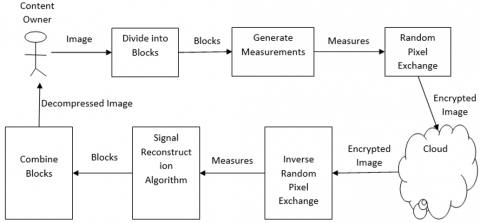Figure 1. Cloud Image Security Framework (CISF)

## 2.png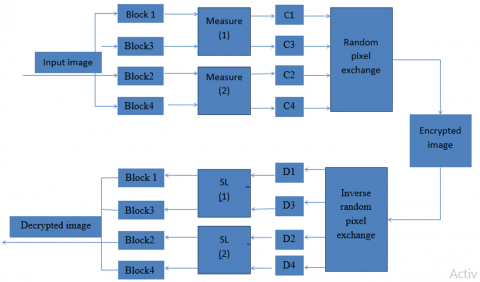Figure 2. Overview the proposed algorithm

4.2 Algorithm design

The prosed algorithm known as Hybrid Image Security Algorithm (HISA) is based on the mechanisms illustrated in Figure 2. The given input image is divided into 4 blocks. First two blocks are used by measure 1 and the remaining are taken by measure 2. The first measure generates two matrices that are subjected to random pixel exchange prior to sending to cloud. The second measure also generates two matrices that are subjected to random pixel exchange prior to sending to cloud. The first measure considers a half part of the image and second measure handles the other part of the image. After this, the reconstruction of original image has reverse process. First, it uses inverse random pixel exchange mechanism in order to produce 4 matrices. Then first two and then second two matrices are used by the signal reconstruction algorithm for generating the 4 blocks originally used. From the blocks, the original image is constructed as final output.

An algorithm is proposed to achieve this mechanism. Hybrid Image Security Algorithm (HISA) is defined based on the illustration provided in Figure 1. It takes an image as input and produces the output image after compression and encryption with the mechanisms such as compressive sensing for concurrent compression and encryption besides using chaotic maps and exchanging pixels in encrypted blocks randomly for additional layer of security.

Algorithm 1: Hybrid Image Security Algorithm

 Algorithm: Hybrid Image Security Algorithm Input: ImageX Output: compressed and encrypted image with compressive sensingX’ Start Divide X into b1, b2, b3, b4 (blocks) Construct two measurement matrices m1, m2 with keys Measure b1 and b3 with m1 Measure b2 and b4 with m2 Step 4 generates C1 and C3 (measurements) Step 5 generates C2 and C4 Map b1, b2, b3, b4 measurements to R2, R1, R2 and R1 Use R1 and R2 to exchange pixels randomly with adjacent blocks Exchange occurs between b1 and b4 Exchange occurs between b2 and b3 Assign outcome to X’ Return X’ End

As presented in Algorithm 1, the input image is converted into an encrypted image after multiple transformations. In the process, the logistic map is used. It is actually constructed by generating a sequence and using it with an iterative process shown in Eq. (8).

$\emptyset(i, 1)=\lambda \emptyset(i-1, N)$

$\emptyset(i, 2: N)=\lambda \emptyset(i-1,1: N-1)$    (8)

The algorithm considers an image with equal height and width as input that is N x N. Divide the image into 4 blocks in such a way that each block is of (N/2 x N/2) size. Then two measurement matrices are generated to handle two blocks each for compression and encryption. Before actually sending the image to cloud, the encrypted image blocks are subjected to random pixel exchange between two adjacent blocks (b1 and b4; b2 and b3). The image reconstruction process by taking outsourced image from cloud is the exact reverse process. However, in order to overcome the problem mentioned in Section 3, we used the SL0 algorithm for reconstruction process.

4.3 Evaluation

Different evaluation metrics are used to know the performance of the proposed framework. Correlation is an important metric used. Correlation of two adjacent pixels in a good quality image is close to 1 while that of encrypted image should be close to 0. Therefore, correlation coefficient reveals the performance of the proposed method. It is computed as in Eq. (9).

$C=\frac{\sum_{i=1}^{N}\left(x_{i}-\bar{x}\right)\left(y_{i}-\bar{y}\right)}{\sqrt{\left(\sum_{i=1}^{N}\left(x_{i}-\bar{x}\right)^{2}\right)\left(\sum_{i=1}^{N}\left(y_{i}-\bar{y}\right)^{2}\right)}}$    (9)

There are three directions used for correlation coefficient computation. They are known as horizontal, vertical and diagonal. An encryption algorithm is actually sensitive to keys. It does mean that a small change in key leads to great impact on the encrypted image. This is measured with mean square error (MSE) as in Eq. (10).

$M S E=\frac{1}{L \times H} \sum_{x y}[I(x, y)-D(x, y)]^{2}$,    (10)

where, the image pixels (total number) are denoted as L x H. The input and output images are denoted s I(x,y) and D(x,y) respectively. Another performance metric used in this paper is PSNR which is widely used for knowing compression performance. It is shown in Eq. (11).

$P S N R$

$=10 \log \frac{255^{2}}{\left(1 / N^{2}\right) \sum_{i}^{N}=1 \sum_{j}^{N}=1[R(i, j)-1(i, j)]^{2}}$    (11)

It is used to find quality of decrypted images with various compression ratios. By measuring PSNR the quality of the proposed encryption technique can be estimated.

5. Results and Discussion

The proposed algorithm named Hybrid Image Security Algorithm (HISA) is evaluated with a prototype application developed in Python data science platform. As presented in Figure 3, Lena is the input image in grey scale with 256x256 resolution. Lena image served as input image (plain image) and each block is of 128x128 size. Compression and encryption results are shown besides decrypted Lena image. The compression ratio used in 4/3 and the key length considered is 2. However, MxN is the key length if the keys are made up of whole measurement matrices which, for example, 192 x 256 leading to large key space. Therefore, in this paper, it is greatly reduced. The parameters used in the empirical study are as follows. M is taken as 96 that is (3/4 x N/2) such that it satisfies $1 \leq M, 1 \leq n \leq N$, λ value is 2, μ value is 3.99 and x01 and x02 values are 0.11 and 0.23 respectively.

## 3.png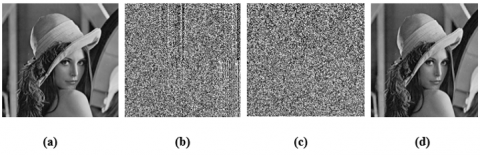Figure 3. Experimental results with Lena image, (a) original image, (b) compressed image, (c) encrypted image, (d) decrypted image

The compressed Lena image is shown in Figure 3 (b) while Figure 3 (c) shows encrypted image and Figure 3 (d) the decrypted image. The proposed algorithm needed less computational operations. Float number addition, multiplication and shift operations are made 512, 704 and 192 times respectively which is very less when compared to the state of the art. The proposed algorithm needed swap operations 256 x 256 times and comparison operations in the random pixel exchange needed 256 x 256 times. The computational complexity of the HISA is significantly less.

Histogram of Lena image is presented in Figure 4 to analyse image encryption performance. When histogram of encrypted image shows values in uniform distribution, it reflects good performance. Figure 4 (a) and Figure 4 (b) show histogram of Lena and its encrypted image respectively. It is found from empirical study that histograms of different input images are similar in order to confuse attackers.

As presented in Figure 5, the correlation distribution of pixels in Lena image and its encrypted counterpart are shown. Correlation shown relation between two adjacent pixels and 1 indicates higher level of correlation and 0 indicates least correlation. Eq. (9) is used in order to perform correlation measure by using 16000 randomly selected adjacent pixels from both images. Least correlation between adjacent pixels shown in Figure 5 (b) reflects performance of the proposed method.

## 4.png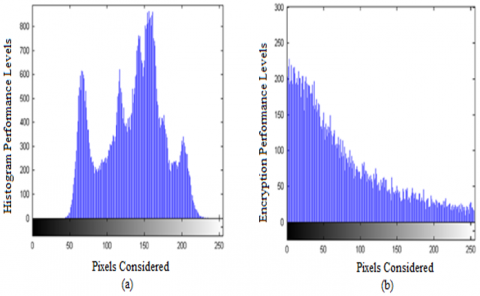Figure 4. Histogram of original Lena image (a) and encrypted Lena image (b)

## 5.png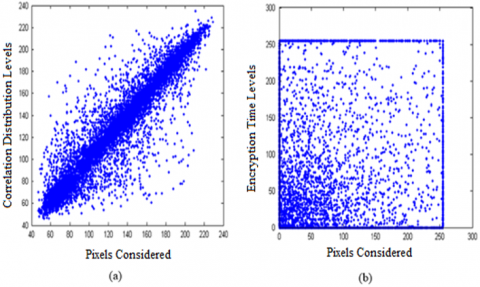Figure 5. Correlation distribution of original Lena image (a) and encrypted image (b)

Table 1. Correlation values for original Lena and encrypted Lena

 Input Images used for Correlation Horizontal Pixel Correlation Vertical Pixel Correlation Diagonal Pixel Correlation Lena Image 0.959959 0.922622 0.908007 Encrypted Lena Image 0.084592 0.058294 0.093091

## 6.pngFigure 6. MSE curve for two decrypted samples

Table 1 shows correlation statistics. The correlation of encrypted Lena is close towards 0 while the correlation of Lena original image is close towards 1 reflecting higher level of performance of the proposed algorithm as it destroys close relationships between adjacent pixels to ensure increased security to media objects. The correlation analysis results also reveal that attackers cannot gain any useful information with attacks based on statistical analysis.

As presented in Figure 6, there are MSE curves for two decrypted images. It is used to analyse key sensitivity which has relation with distortion of decrypted images. Image encryption algorithms are sensitive to keys therefore; this analysis assumes significance. Eq. (10) is used for MSE computations. Great distortion shown in the decrypted images as in Figure 6 (a) and Figure (b) reflect the efficiency of the proposed algorithm which is sensitive to keys and the key space is reduced in this experiment to reap its benefits.

As presented in Figure 7, PSNR measure (as per Eq. (11)) is used to know compression performance of the proposed algorithm that is capable of compressing and encrypting simultaneously. Higher PSNR indicates good performance. From the results it revealed that with all given compression ratio, the HISA has shown better performance in terms of quality of decrypted images.

In addition to safety concern, it is also important to be able to survive on these attacks for the image encryption framework in the light of the variable tolerance of image processing operations such as noise addition or cutting, image compression, etc. This paper is used to analyse the visual output of the decrypted image I' in contrast to the single image I by PSNR (Peak Signal-to-Noise Ratio.

The hybrid approach has provided performance improvement. It is also verified against noise attacks. When Gaussian noise is added to encrypted image, the proposed algorithm is still able to provide acceptable performance in terms of quality of decrypted image. When compared with state of the art where whole measurement matrix is used as key, the proposed methods reduces key space greatly and that has influence on performance enhancement. Security is further enhanced by using random pixel exchange employed as the process of the HISA. The experimental results revealed that HISA achieves improved performance in terms of compression and security to digital objects.

## 7.pngFigure 7. PSNR for Lena decrypted image with different compression ratios

6. Conclusion and Future Work

In this paper, we proposed a framework known as Cloud Image Security Framework (CISF) leveraging outsourced image security. The framework has an underlying algorithm named Hybrid Image Security Algorithm (HISA). It is based on compressive sensing, simultaneous sensing and encryption besides random pixel exchange to ensure multiple transformations of input image. The given image is compressed and encrypted before outsourcing it to cloud. It has multiple transformations in order to ensure high level of protection. In the process compressive sensing and random pixel exchange are used. Randomly exchanging pixels after performing encryption adds another layer of security to the compressed and encrypted image. Another important contribution of this paper is reduction of key size which has significance in many real time applications in distributed environments. Empirical study is made with a prototype made using Python data science platform. The experimental results revealed that the proposed framework is useful and can help data owners or multimedia content providers to securely outsource their intellectual property to public cloud. With high level of security and increased compression performance, the results have given impetus to further investigations. In future we enhance our framework to consider requirements pertaining to secure deduplication.

References

 Kumar, M.T., Reddy, M.B. (2017). Compressive sensing based simultaneous data compression and convergent encryption for secure deduplication. IJCSIS, 15(9): 144-147.

 Wang, L., Li, L., Li, J., Li, J., Gupta, B.B., Liu, X. (2018). Compressive sensing of medical images with confidentially homomorphic aggregations. IEEE Internet of Things Journal, 6(2): 1402-1409. https://doi.org/10.1109/JIOT.2018.2844727

 Hsieh, S.H., Hung, T.H., Lu, C.S., Chen, Y.C., Pei, S.C. (2018). A secure compressive sensing-based data gathering system via cloud assistance. IEEE Access, 6: 31840-31853. https://doi.org/10.1109/ACCESS.2018.2844184

 Dawood, Q.M., Rao, K.G., Rao, B.B. (2018). Secure video data deduplication in the cloud storage using compressive sensing. IJCST, 6: 38-46.

 Hu, G., Xiao, D., Xiang, T., Bai, S., Zhang, Y. (2017). A compressive sensing based privacy preserving outsourcing of image storage and identity authentication service in cloud. Information Sciences, 387: 132-145. https://doi.org/10.1016/j.ins.2016.09.045

 Yu, J., Guo, S., Song, X., Xie, Y., Wang, E. (2020). Image parallel encryption technology based on sequence generator and chaotic measurement matrix. Entropy, 22(1): 76. https://doi.org/10.3390/e22010076

 Usama, M., Zakaria, N. (2017). Chaos-based simultaneous compression and encryption for Hadoop. PloS One, 12(1): e0168207. https://doi.org/10.1371/journal.pone.0195420

 Kandasamy, V., Siva Alagesh, S., Pradeepraj, C. (2018). Data protection with de-duplication in cloud computing. International Journal of Science, Engineering and Technology Research (IJSETR), 7(4): 188-191.

 Karthika, R.N., Valliyammai, C., Abisha, D. (2017). Perlustration on techno level classification of deduplication techniques in cloud for big data storage. In 2016 Eighth International Conference on Advanced Computing (ICoAC), pp. 206-211. https://doi.org/10.1109/ICoAC.2017.7951771

 Ji, S., Tan, C., Yang, P., Sun, Y.J., Fu, D., Wang, J. (2018). Compressive sampling and data fusion-based structural damage monitoring in wireless sensor network. The Journal of Supercomputing, 74(3): 1108-1131. https://doi.org/10.1007/s11227-016-1938-x

 Rahman, M.T., Salan, M.S.A., Shuva, T.F., Khan, R.T. (2017). Efficient sensor-cloud communication using data classification and compression. International Journal of Information Technology and Computer Science (IJITCS), 9(6): 9-17.

 Luo, E., Bhuiyan, M.Z.A., Wang, G., Rahman, M.A., Wu, J., Atiquzzaman, M. (2018). Privacyprotector: Privacy-protected patient data collection in IoT-based healthcare systems. IEEE Communications Magazine, 56(2): 163-168. https://doi.org/10.1109/MCOM.2018.1700364

 Yang, C., Huang, Q., Li, Z., Liu, K., Hu, F. (2017). Big Data and cloud computing: Innovation opportunities and challenges. International Journal of Digital Earth, 10(1): 13-53. https://doi.org/10.1080/17538947.2016.1239771

 Ajdari, M., Lee, W., Park, P., Kim, J., Kim, J. (2019). FIDR: A scalable storage system for fine-grain inline data reduction with efficient memory handling. In Proceedings of the 52nd Annual IEEE/ACM International Symposium on Microarchitecture, pp. 239-252. https://doi.org/10.1145/3352460.3358303

 Tang, Y., Li, D., Li, Z., Zhang, M., Jee, K., Xiao, X., Li, Q. (2018). Nodemerge: Template based efficient data reduction for big-data causality analysis. In Proceedings of the 2018 ACM SIGSAC Conference on Computer and Communications Security, pp. 1324-1337. https://doi.org/10.1145/3243734.3243763

 Dai, H.N., Wong, R.C.W., Wang, H., Zheng, Z., Vasilakos, A.V. (2019). Big data analytics for large-scale wireless networks: Challenges and opportunities. ACM Computing Surveys (CSUR), 52(5): 1-36. https://doi.org/10.1145/3337065

 Lytvyn, V., Vysotska, V., Osypov, M., Slyusarchuk, O., Slyusarchuk, Y. (2019). Development of intellectual system for data de-duplication and distribution in cloud storage. Webology, 16(2): 1-42.

 Wu, S., Chen, X., Mao, B. (2016). Exploiting the data redundancy locality to improve the performance of deduplication-based storage systems. In 2016 IEEE 22nd International Conference on Parallel and Distributed Systems (ICPADS), pp. 527-534. https://doi.org/10.1109/ICPADS.2016.0076

 Mohimani, H., Babaie-Zadeh, M., Jutten, C. (2008). A fast approach for overcomplete sparse decomposition based on smoothed ℓ0 norm. IEEE Transactions on Signal Processing, 57(1): 289-301. https://doi.org/10.1109/TSP.2008.2007606